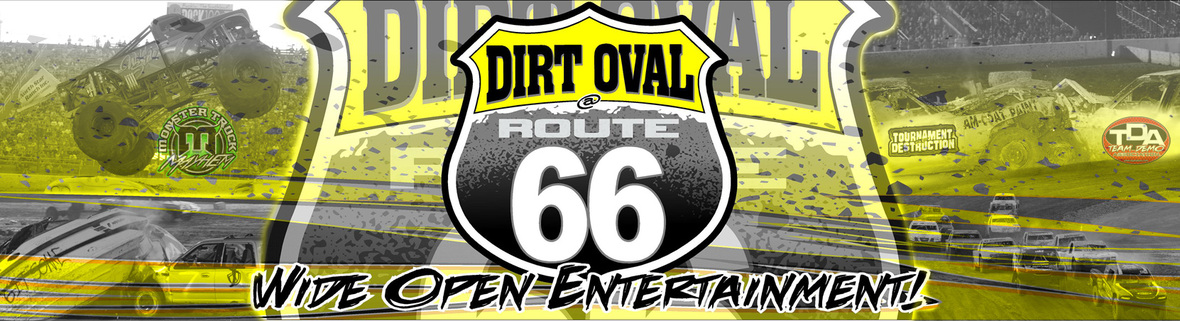On a Collision Course to the Team Demolition Derby Championship

 table.module-2{width:96.6%;padding:0}table div table+table+table div table{width:96.6%;float:none;margin-left:auto;margin-right:auto;padding:0}table div table+table+table div table a{border:0 none;text-decoration:none}table div table+table+table div table img{width:100%!important;border:0 none;text-decoration:none}table div table+table+table div table td{width:100%;padding:0}/* styles */

The most Impactful and Important Race of the Season!

The 2017 Championship hangs in the balance. Only 7 points separate all 8 teams with just 2 events remaining. Orange Crush, Crazy 8s and Mean Green Machine have scored victory thus far. Can a fourth team smash through the competition and completely shake up the points?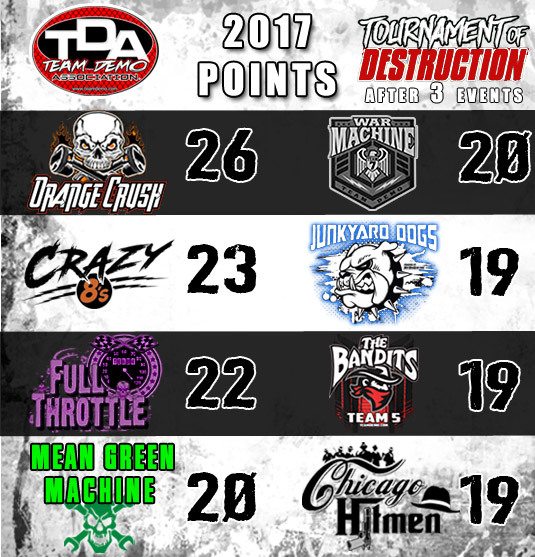Mean Green Machine is back on the attack and ready to uphold their history of Round 4 dominance. Five of the last seven Round 4 event wins belong to Mean Green Machine.
But after sitting out Round 3 last month, are they still at Championship form?

War Machine, Orange Crush, Full Throttle or Crazy 8s will be ready to greet them with a rude awakening in colossal first round slugfest Saturday night!

 /* styles */ 4 WAYS TO WATCH IT LIVE: Buy now @ TeamDemo.com Call 815-740-8000 At the Dirt Oval office On Race Day at the gate Zero processing fees with in-person purchase. Kids 2yrs & under are FREE. Call us about group discounts.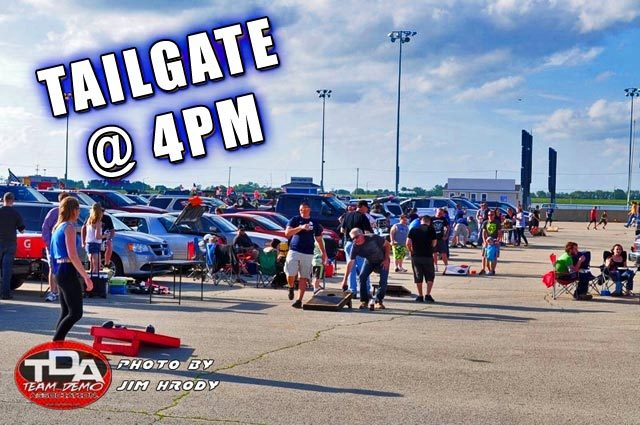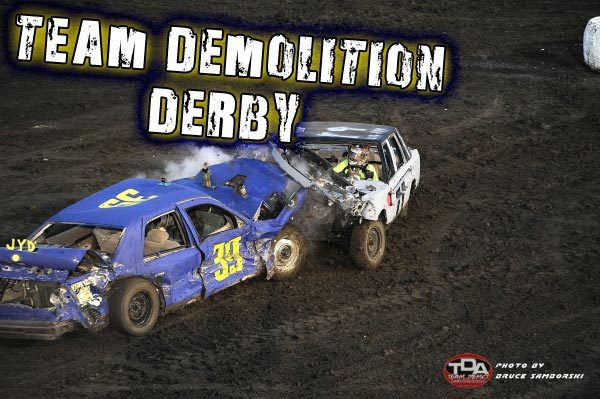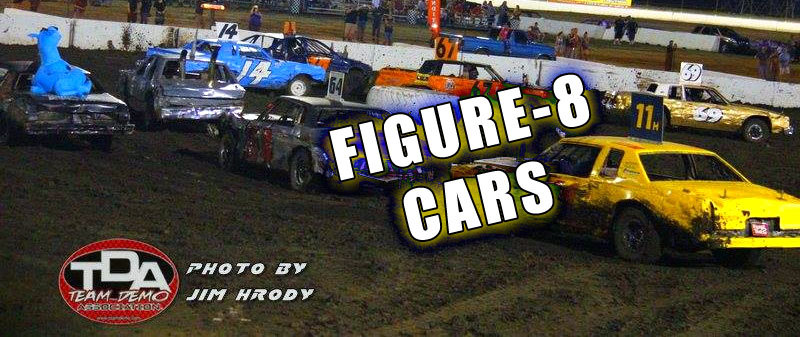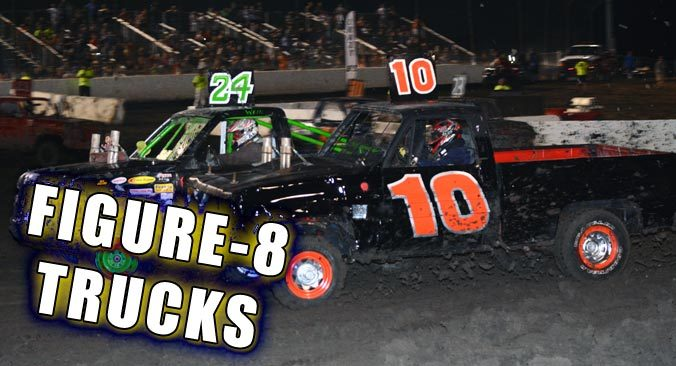table.module-10{width:95.66%;padding:0}table div table+table+table+table+table+table+table+table+table+table+table div table{width:95.66%;float:none;margin-left:auto;margin-right:auto;padding:0}table div table+table+table+table+table+table+table+table+table+table+table div table a{border:0 none;text-decoration:none}table div table+table+table+table+table+table+table+table+table+table+table div table img{width:100%!important;border:0 none;text-decoration:none}table div table+table+table+table+table+table+table+table+table+table+table div table td{width:100%;padding:0}/* styles */

Full weekend of Scout activities begins FridayHundreds of Boy & Girl Scouts converge for largest gathering at the Dirt Oval to date!

Scouts will stake their tents in the shadow of the Dirt Oval for a full weekend of fun leading up to Tournament of Destruction Round 4 Saturday Night!
Obstacle Course | Automotive Maintenance | Car Painting | Campfires | and more unique experiences for all ages.

Thank You to all the Troops and Scouts for their participation, efforts and excitement which has transformed the event into a full weekend and drawn in Troops from throughout the Midwest.

Schedule of Events for Scout Troops

 table.module-15{width:87.55%;padding:0}table div table+table+table+table+table+table+table+table+table+table+table+table+table+table+table+table div table{width:87.55%;float:none;margin-left:auto;margin-right:auto;padding:0}table div table+table+table+table+table+table+table+table+table+table+table+table+table+table+table+table div table a{border:0 none;text-decoration:none}table div table+table+table+table+table+table+table+table+table+table+table+table+table+table+table+table div table img{width:100%!important;border:0 none;text-decoration:none}table div table+table+table+table+table+table+table+table+table+table+table+table+table+table+table+table div table td{width:100%;padding:0}/* styles */
 /* styles */ Property Map (.PDF) | Directions to Track
 /* styles */ Contact Samantha@dirtoval66.com or call 815-740-8000 with any last-minute questions. Any employee wearing a bright yellow TEAM DIRT shirt can answer questions on-site throughout the weekend.
 table.module-18{width:86.98%;padding:0}table div table+table+table+table+table+table+table+table+table+table+table+table+table+table+table+table+table+table+table div table{width:86.98%;float:none;margin-left:auto;margin-right:auto;padding:0}table div table+table+table+table+table+table+table+table+table+table+table+table+table+table+table+table+table+table+table div table a{border:0 none;text-decoration:none}table div table+table+table+table+table+table+table+table+table+table+table+table+table+table+table+table+table+table+table div table img{width:100%!important;border:0 none;text-decoration:none}table div table+table+table+table+table+table+table+table+table+table+table+table+table+table+table+table+table+table+table div table td{width:100%;padding:0}/* styles */

Fun in the MUD next Weekend!

 table div table+table+table+table+table+table+table+table+table+table+table+table+table+table+table+table+table+table+table+table+table div table td,table.module-20{width:100%;padding:0}table div table+table+table+table+table+table+table+table+table+table+table+table+table+table+table+table+table+table+table+table+table div table{width:100%;float:none;margin-left:auto;margin-right:auto;padding:0}table div table+table+table+table+table+table+table+table+table+table+table+table+table+table+table+table+table+table+table+table+table div table a{border:0 none;text-decoration:none}table div table+table+table+table+table+table+table+table+table+table+table+table+table+table+table+table+table+table+table+table+table div table img{width:100%!important;border:0 none;text-decoration:none}/* styles */
 /* styles */ Bring your 4x4 and your Redneck-side to the Inaugural Redneck Jamboree at The Dirt Oval. Live Music all day | Mega Trucks | Tug-o-Wars | Burnouts Driver/Competitor registration is on-site only. All Access passes are \$20 thru Aug 27th | \$25 day-of. Contact the Dirt Oval 66 ticket office for your pass! 815-740-8000 | info@dirtoval66.com

Dirt before NASCAR! Chicagoland's "Dirty 30" launches Four-Race Weekend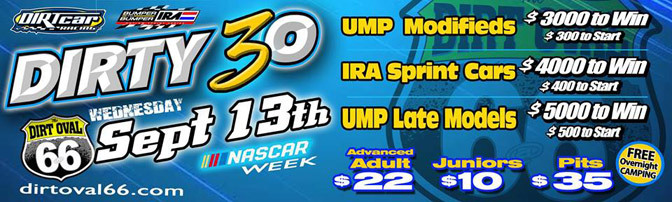5 Races. 2 Tracks. Zero Reason to miss out!

All kickin' up dust on the 3/8 Mile Dirt Oval on Wednesday ahead of NASCAR at Chicagoland Speedway Thurs - Sunday.

FREE Overnight Camping
for Dirty 30! Call 815-740-8000
to reserve your spot.

 table.module-25{width:91.7%;padding:0}table div table+table+table+table+table+table+table+table+table+table+table+table+table+table+table+table+table+table+table+table+table+table+table+table+table+table div table{width:91.7%;float:none;margin-left:auto;margin-right:auto;padding:0}table div table+table+table+table+table+table+table+table+table+table+table+table+table+table+table+table+table+table+table+table+table+table+table+table+table+table div table a{border:0 none;text-decoration:none}table div table+table+table+table+table+table+table+table+table+table+table+table+table+table+table+table+table+table+table+table+table+table+table+table+table+table div table img{width:100%!important;border:0 none;text-decoration:none}table div table+table+table+table+table+table+table+table+table+table+table+table+table+table+table+table+table+table+table+table+table+table+table+table+table+table div table td{width:100%;padding:0}/* styles */

Team Demo Derby #throwbackthursday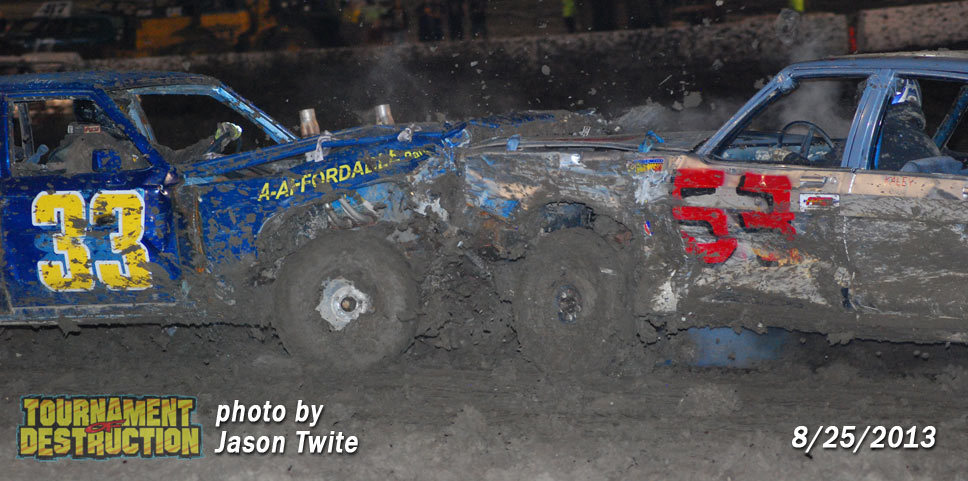/* styles */ The importance of winning Round 4 is no secret. Mean Green Machine, Real Steel and Junkyard Dogs are proof of the wide-range of affects just one night; just one race can have on a season. Orange Crush, Junkyard Dogs and Real Steel won the first three events in 2013; much like the current trend in 2017. Real Steel prevented a fourth team from entering the Championship picture by defeating Full Throttle, JYD and then MGM in the Finals. The loss knocked MGM from contention and put Real Steel in a 1st place deadlock with Crush. JYD played a key part in that puzzle thanks to eliminating OC early in the bracket. Although they lost to Real Steel in the semi-Finals, JYD gained 1-point overall, which setup a 3-way battle for the ages at Round 5. side-note: Junkyard Dogs vs. Orange Crush is easily Top 5... probably Top 3... and perhaps the greatest Team Demo race ever at Dirt Oval 66.
 table.module-29{width:75.47%;padding:0}table div table+table+table+table+table+table+table+table+table+table+table+table+table+table+table+table+table+table+table+table+table+table+table+table+table+table+table+table+table+table div table{width:75.47%;float:none;margin-left:auto;margin-right:auto;padding:0}table div table+table+table+table+table+table+table+table+table+table+table+table+table+table+table+table+table+table+table+table+table+table+table+table+table+table+table+table+table+table div table a{border:0 none;text-decoration:none}table div table+table+table+table+table+table+table+table+table+table+table+table+table+table+table+table+table+table+table+table+table+table+table+table+table+table+table+table+table+table div table img{width:100%!important;border:0 none;text-decoration:none}table div table+table+table+table+table+table+table+table+table+table+table+table+table+table+table+table+table+table+table+table+table+table+table+table+table+table+table+table+table+table div table td{width:100%;padding:0}/* styles */
 table div table+table+table+table+table+table+table+table+table+table+table+table+table+table+table+table+table+table+table+table+table+table+table+table+table+table+table+table+table+table+table div table{width:100%;padding:0}table div table+table+table+table+table+table+table+table+table+table+table+table+table+table+table+table+table+table+table+table+table+table+table+table+table+table+table+table+table+table+table div table table{padding:0;float:left!important;width:48.111%!important}table div table+table+table+table+table+table+table+table+table+table+table+table+table+table+table+table+table+table+table+table+table+table+table+table+table+table+table+table+table+table+table div table td{padding-left:13px;padding-right:13px}table div table+table+table+table+table+table+table+table+table+table+table+table+table+table+table+table+table+table+table+table+table+table+table+table+table+table+table+table+table+table+table div table table td{padding-left:0;padding-right:20px}table div table+table+table+table+table+table+table+table+table+table+table+table+table+table+table+table+table+table+table+table+table+table+table+table+table+table+table+table+table+table+table div table table+table{float:left!important;width:51.889%!important}table div table+table+table+table+table+table+table+table+table+table+table+table+table+table+table+table+table+table+table+table+table+table+table+table+table+table+table+table+table+table+table div table table+table td{padding-left:0;padding-right:0}/* styles */
 Like   Tweet